Name:    Chapter 1 Post Test

Multiple Choice
Identify the choice that best completes the statement or answers the question.

1.

Plot the number 2.8 on the real number line.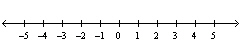a.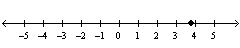b.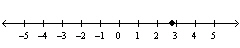c.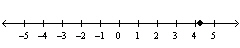d.e.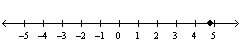2.

Find a true inequality that involves the real number.
 a.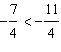b.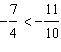c.d.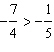e.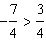3.

Find all real numbers whose distance from –7 is 3.
 a. –10 b. 10 c. –10 and –4 d. –4 e. 10 and –4

4.

Find the sum.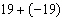a. 0 b. 2 c. –2 d. –1 e. 1

5.

Find the sum..
 a. 28,257 b. 18,791 c. –15,385 d. –19,403 e. 22,809

6.

Find the difference.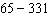a. –276 b. 276 c. –256 d. –266 e. 266

7.

Find the product.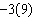a. 12 b. –27 c. 6 d. –12 e. 27

8.

Find the product.a. 5 b. 144 c. –1152 d. 1152 e. –144

9.

Use the long division algorithm to find the quotient.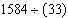a. 48 b. 33 c. 43 d. 53 e. 38

10.

Write the prime factorization of the number 1485.
 a.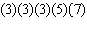b.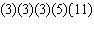c.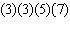d.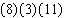e.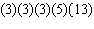11.

An object moves a distance of 490 miles in 7 hours. What is the object's average speed in miles per hour?
 a.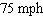b.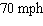c.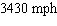d.e.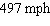12.

Find the volume of the rectangular solid.10 in. 8 in.4 in.
 a.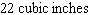b.c.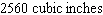d.e.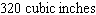13.

Subtract the fractions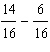and write the result in simplest form.
 a.b.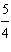c.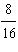d.e.14.

Determine the unknown fractional part of the circle graph.a.b.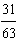c.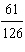d.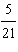e.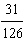15.

Multiplyand write the result in simplest form.
 a.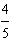b.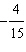c.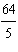d.e.16.

Evaluate the expression, if possible.
 a.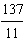b.c.d.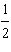e. not possible

17.

Identify the property of real numbers illustrated by the statement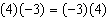.
 a. Commutative Property of Multiplication b. Commutative Property of Addition c. Distributive Property d. Associative Property of Addition e. Associative Property of Multiplication

18.

Find the additive inverse and the multiplicative inverse of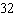.
 a. additive inverse: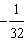multiplicative inverse: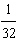b. additive inverse:multiplicative inverse:c. additive inverse:multiplicative inverse:d. additive inverse:multiplicative inverse:e. additive inverse:multiplicative inverse:19.

Simplify the expression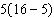using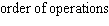.
 a.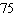b.c.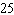d.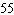e.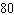20.

Write an expression for the perimeter of the triangle shown in the following figure.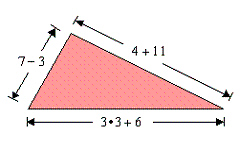a.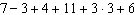b.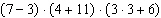c.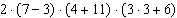d.e.